# OT和OT-Extension

Posted by 王宗岳 on 11-03，2021

## OT

OT 是双方的交互协议，交互双方为Sender和Receiver。Sender有两个消息${m_0, m_1}$，Receiver选择$b\in\{0,1\}$，完成Sender将$m_b$发送给Receiver。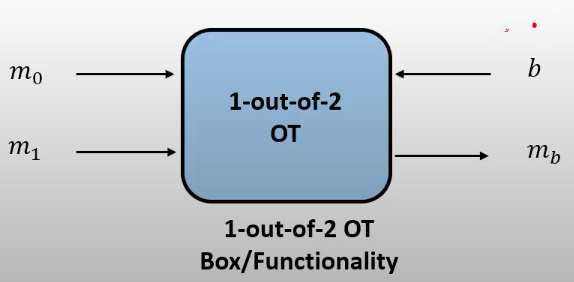• Rabin's OT Sender有1个消息m，Receiver以$\frac{1}{2}$的概率获得m或者噪声；
• 1 out of N OT Sender有$N$个消息，Receiver选择$b\in\{0,1,\cdots N\}$；
• k out of N OT Sender有$N$个消息，Receiver选择${b_1, b_2, \cdots b_k }, b_i \in \{0,1,\cdots N\}$；

• Sender和Receiver完成两个1 out of 2 OT，传递$\{k_0, k_1\}$和${k_{0}', k_{1}'}$，Reciver选择$b_0, b_1$拿到$k_{b_0}$和$k_{b_1}$;
• Sender将${m_0,m_1,m_2,m_3}$加密到${c_0,c_1,c_2,c_3}$，分别使用密钥$\{[k_0,k_{0}'], [k_0,k_{1}'],[k_1,k_{0}'],[k_1,k_{1}']\}$。加密可以简单使用$c_0 = m_0 \oplus F_{k_0}(00)) \oplus F_{k_{0}'}(00)$，$c_1 =m_1 \oplus F_{k_0}(01))\oplus F_{k_{1}'}(01)$以此类推。这里不能简单的使用$\oplus k_i$，因为这样Receiver可获得$c_0\oplus c_1\oplus c_2\oplus c_3 = m_0\oplus m_1\oplus m_2\oplus m_3$，有信息泄漏;
• Sender将${c_0,c_1,c_2,c_3}$发送给Receiver，则Receiver只能解密$b_0 b_1$对应密文；

## OT 的构造

OT可以通过Diff-Hellman进行构造，如下所示：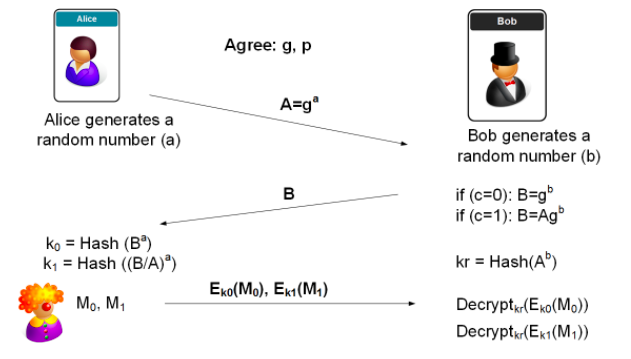Bob 作为Receiver，选择c，Alice 作为sender，发送$M_0$或$M_1$

## OT Extension

Sender和Receiver希望进行 $k$ 次OT，每次传递$l$ bit 的消息，通过 $k$ 次$l$ bit 的 OT，可以完成。而OT Extension可以大大增大传输效率。通过OT Extension，可以仅进行 $\lambda$ 次 $k$ bit的OT，就可以实现。该论文发表在CRYPTO 2003,"Extending Oblivious Transfer Efficiently".

### magic 方法

1. 首先假设有一种 magic 方法，可以实现以下结果：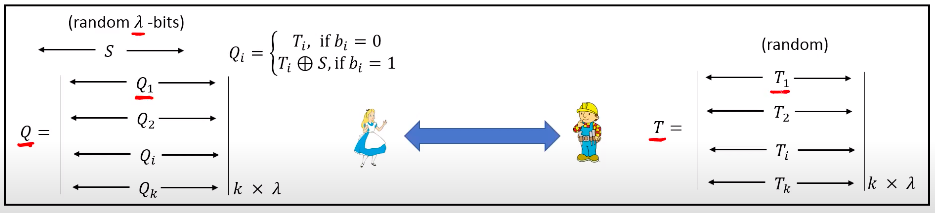• Alice (Sender) 选择一个随机的长度为 $\lambda$ 的数 $S$
• Bob (Receiver) 随机生成一个 $k$ 行， $\lambda$ 列的矩阵 $T$，每一个元素均为1 bit
• 通过 magic 方法，Alice 可以拿到矩阵 $Q$，其中，根据 Bob 的选择
$\{b_0,b_1,\cdots b_k\}$, $b_i \in \{0, 1\}$
$$Q_i= \begin{cases} T_i,\quad &if \quad b_i=0\\ T_i\oplus S,\quad & if \quad b_i=1 \end{cases}$$
1. 通过这个 magic 方法，Alice 可将消息对 $m_, m_$ 使用 $m_ \oplus H(i, Q_i)$ 和 $m_ \oplus H(i, Q_i\oplus S)$ 进行加密，$Q_i$ 和 $Q_i\oplus S$ 中有一个是$T_i$，而Bob不知道 $S$，所以只能恢复他选择的这个消息。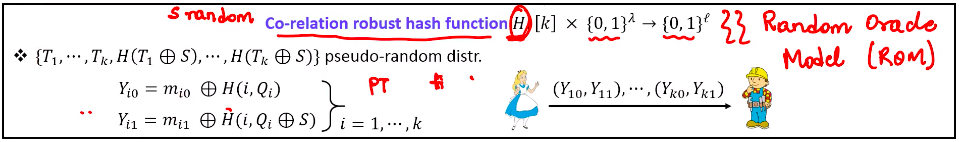这样就完成了$k$ 次，$l$ bit 的消息的OT。

### 如何实现 magic 方法

1. Bob生成矩阵$T$，Alice生成$S$
2. Bob 作为 Sender，Alice 作为Receiver，完成 $\lambda$ 次 OT，其中消息Alice根据$s_i$选择接受$T^i$或者$T^i\oplus B$，$B$为Bob的选择向量。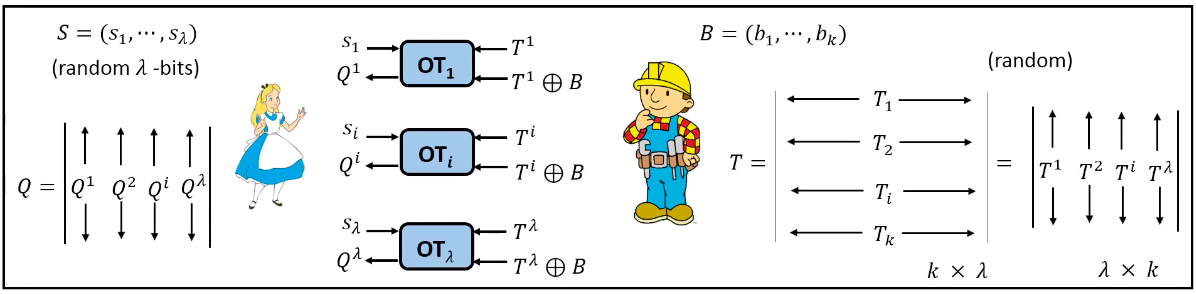这样就完成了magic 方法，可以按下图进行分析：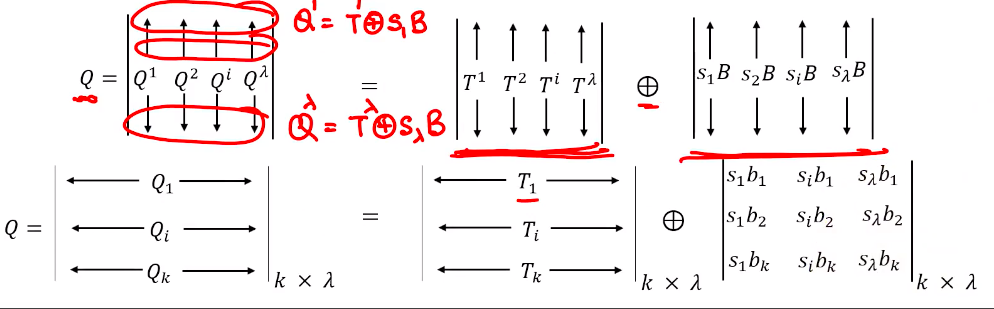## 参考视频